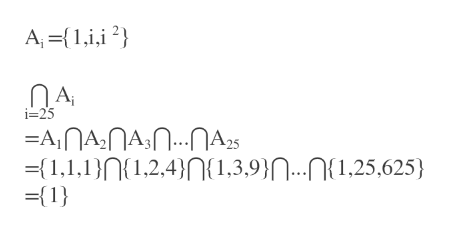Use the following definitions to express each union or intersection given. You can use roster or set builder notation in your responses, but no set operations.      Ai={i0,i1,i2}(Recall that for any numberx, x0=1.)        Bi={x∈R:−i≤x≤1/i}         Ci={x∈R:−1/i≤x≤1/i}(a)   ⋂i=25Ai(b)   ⋃i=25Ai(c)    ⋂i=1100Bi(d)    ⋃i=1100Bi(e)    ⋂i=1100Ci(f)    ⋃i=1100Ci

Question

Use the following definitions to express each union or intersection given. You can use roster or set builder notation in your responses, but no set operations.

Ai={i0,i1,i2}
(Recall that for any number

x
,

x0=1
.)

Bi={x∈R:−i≤x≤1/i}

Ci={x∈R:−1/i≤x≤1/i}
(a)

⋂i=25Ai
(b)

⋃i=25Ai
(c)

⋂i=1100Bi
(d)

⋃i=1100Bi
(e)

⋂i=1100Ci
(f)

⋃i=1100Ci

Step 1

Since we only answer up to 3 sub-parts, we’ll answer the first 3. Please resubmit the question and specify the other subparts (up to 3) you’d like answered.

a. Ai={i^0,i^1,i^2}

Ai= {1,i,i^2}help_outlineImage TranscriptioncloseA, {1 2 i=25 -AnAAnA2 [1,1,n2,4{1,3,9}|...N(1,25,625) [1 fullscreen
Step 2

Want to see the full answer?

See Solution

Want to see this answer and more?

Our solutions are written by experts, many with advanced degrees, and available 24/7

See Solution
Tagged in

Algebra